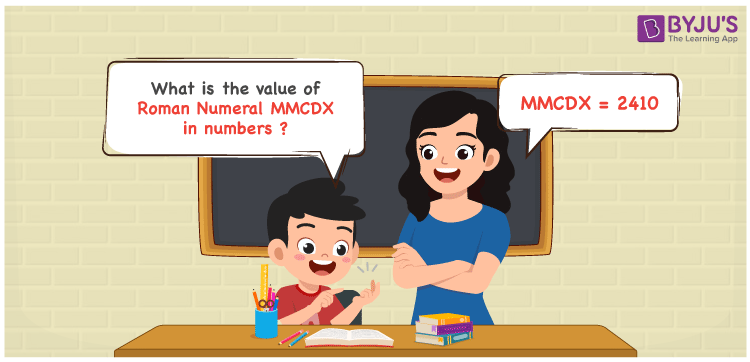Checkout JEE MAINS 2022 Question Paper Analysis : Checkout JEE MAINS 2022 Question Paper Analysis :

# MMCDX Roman Numerals

MMCDX Roman Numerals is 2410. This article discusses in detail on how the roman numerals MMCDX is written in numbers in an understanding way. If these fundamentals are learnt well, curating perfect answers in the exams will be very easy. This roman numerals conversion article is the best suited material for the exam preparation of primary students. Therefore, MMCDX can be indicated as 2410 in numbers.

 Number Roman Numeral 2410 MMCDX## How to Write MMCDX Roman Numerals in Numbers?

Students will know how MMCDX is written in numbers using the steps below.

MMCDX = M + M + (D – C) + X

MMCDX = 1000 + 1000 + (500 – 100) + 10

MMCDX = 2410

## Video Lesson on Roman Numerals## Frequently Asked Questions on MMCDX Roman Numerals

### Why is 2410 written as MMCDX?

In roman numerals

2000 is written as MM

400 is written as CD

10 is written as X

2410 = MM + CD + X = MMCDX

### Find 2500 – 90.

We know that

2500 – 90 = 2410

Hence, 2500 – 90 is 2410 which is written as MMCDX.

### Find the remainder we get when MMCDX is divided by XVI.

We know that

MMCDX = 2410

XVI = 16

So the remainder we get when MMCDX is divided by XVI is X.